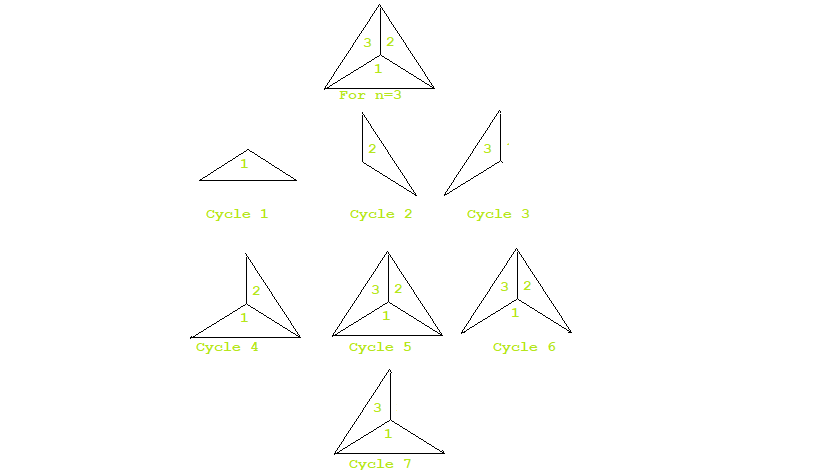# Number of cycles formed by joining vertices of n sided polygon at the center

• Last Updated : 28 Jun, 2022

Given an N-sided regular polygon, we have connected all the vertices at the center of the polygon, thus dividing the polygon into N equal parts. Our task is to the Count of the total number of cycles in the polygon.
Note: A Cycle is a closed-loop starting and ending on same point.
Examples:

Input: N = 3
Output:
Explanation:When a 3 sided polygon is connected by vertices at the center then we get 7 cycles possible for it as shown in the image.
Input: N = 5
Output: 21
Explanation:When a 5 sided polygon is connected by vertices at the center then we get 21 cycles possible for it as shown in the image.

Approach: To the problem mentioned above we are supposed to count the total number of closed loops possible in the given polygon after division. The approach is based upon Mathematical Pattern. There will be N cycles already created due to the division of polygon. One out of N blocks will form a cycle with rest (N – 1) blocks. The remaining (N – 1) blocks will form cycle with other (N – 2) blocks. So the total cycles we have can be found out using the formula given below:

Total Cycles = N + 1 * (N – 1) + (N – 1) * (N – 2)
Total Cycles = 2 * N – 1) + (N – 1) * (N – 2)

Below is the implementation of the above approach:

## C++

 `// C++ program for the above approach``#include``using` `namespace` `std;` `// Function to calculate number of cycles``int` `findCycles(``int` `N)``{``    ``int` `res = 0;``    ``int` `finalResult = 0;``    ``int` `val = 2 * N - 1;`` ` `    ``// BigInteger is used here``    ``// if N=10^9 then multiply``    ``// will result into value``    ``// greater than 10^18``    ``int` `s = val;`` ` `    ``// BigInteger multiply function``    ``res = (N - 1) * (N - 2);``    ``finalResult = res + s;`` ` `    ``// Return the final result``    ``return` `finalResult;``}` `// Driver code``int` `main()``{``    ``// Given N``    ``int` `N = 5;`` ` `    ``// Function Call``    ``cout << findCycles(N) << endl;   ``    ``return` `0;``}` `// This code is contributed by divyeshrabadiya07`

## Java

 `// Java program for the above approach` `import` `java.util.*;``import` `java.math.*;` `class` `GFG {` `    ``// Function to calculate number of cycles``    ``static` `BigInteger findCycles(``int` `N)``    ``{``        ``BigInteger res, finalResult;``        ``long` `val = ``2` `* N - ``1``;` `        ``String st = String.valueOf(val);` `        ``// BigInteger is used here``        ``// if N=10^9 then multiply``        ``// will result into value``        ``// greater than 10^18` `        ``BigInteger str = ``new` `BigInteger(st);``        ``String n1 = String.valueOf((N - ``1``));``        ``String n2 = String.valueOf((N - ``2``));` `        ``BigInteger a = ``new` `BigInteger(n1);``        ``BigInteger b = ``new` `BigInteger(n2);` `        ``// BigInteger multiply function``        ``res = a.multiply(b);` `        ``finalResult = res.add(str);` `        ``// Return the final result``        ``return` `finalResult;``    ``}` `    ``// Driver Code``    ``public` `static` `void``    ``main(String args[]) ``throws` `Exception``    ``{``        ``// Given N``        ``int` `N = ``5``;` `        ``// Function Call``        ``System.out.println(findCycles(N));``    ``}``}`

## Python3

 `# Python3 program for the above approach`` ` `# Function to calculate number of cycles``def` `findCycles(N):``    ``res ``=` `0``    ``finalResult ``=` `0``    ``val ``=` `2` `*` `N ``-` `1``;` `    ``# BigInteger is used here``    ``# if N=10^9 then multiply``    ``# will result into value``    ``# greater than 10^18``    ``s ``=` `val` `    ``# BigInteger multiply function``    ``res ``=` `(N ``-` `1``) ``*` `(N ``-` `2``)``    ``finalResult ``=` `res ``+` `s;` `    ``# Return the final result``    ``return` `finalResult;` `# Driver Code``if` `__name__``=``=``'__main__'``:``    ` `    ``# Given N``    ``N ``=` `5``;` `    ``# Function Call``    ``print``(findCycles(N));` `    ``# This code is contributed by pratham76`

## C#

 `// C# program for the above approach``using` `System;``class` `GFG {` `  ``// Function to calculate number of cycles``  ``static` `int` `findCycles(``int` `N)``  ``{``    ``int` `res = 0;``    ``int` `finalResult = 0;``    ``int` `val = 2 * N - 1;` `    ``// BigInteger is used here``    ``// if N=10^9 then multiply``    ``// will result into value``    ``// greater than 10^18``    ``int` `s = val;` `    ``// BigInteger multiply function``    ``res = (N - 1) * (N - 2);``    ``finalResult = res + s;` `    ``// Return the final result``    ``return` `finalResult;``  ``}` `  ``// Driver code``  ``static` `void` `Main()``  ``{``    ` `    ``// Given N``    ``int` `N = 5;` `    ``// Function Call``    ``Console.WriteLine(findCycles(N));``  ``}``}` `// This code is contributed by divyesh072019`

## Javascript

 ``

Output:

`21`

Time Complexity: O(1)
Auxiliary Space: O(1)

My Personal Notes arrow_drop_up